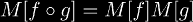# Glossary

 Abel function An Abel function A(z) is a function associated with a function f (z) that satisfies Abel's functional equation:         A( f (z)) = A(z) + 1 Abel matrix (NEW!) -- A matrix based on the Bell matrix. addition Addition is a binary operation defined on the complex numbers. For more information on this website, click here. analytic A special case of continuous meaning infinitely differentiable, plus some other requirements. The other requirements for a function being analytic differ depending on whether the requirements are for real analytic or complex analytic functions. real analytic complex analytic Bell matrix A Bell matrix B[ f ] is a matrix associated with a function f (x) such that:for all g(x). binary operation . Carleman matrix A Carleman matrix M[ f ] is a matrix associated with a function f (x) such that:for all g(x). closed form . continuous Continuous things are usually contrasted with discrete things. A good example of something continuous is the set of real numbers. continuous iteration Continuous iteration is a generalization of discrete iteration. This effectively turns a function f (x) into a function g(n, x) = f n(x). Two ways of doing this are to satisfy:         g(n, x) = f n(x)         for integer n which is a weaker form of continuous iteration, or:         g(1, x) = f (x)                 and         g(y, x) = f (g(y − 1, x))         for all real y, and         g(y, x) = g(y − 1, f (x))         for all real y which is a stronger form of continuous iteration. discrete Discrete things are usually contrasted with continuous things. A good example of something discrete is the set of integers. discrete iteration See iteration dynamical system endofunction The prefix endo- is usually used with terms with a domain and range like functions, morphisms, and functors. An endofunction is a function whose domain and range are the same set. evolution function exponential factorial Not to be confused with ordinary factorials. The exponential factorial is defined by EF(x) = xEF(x-1). For more information on this website click here. For more information from other websites, see Wikipedia. exponential An exponential function is any function of the form:         expb(z) = bz exponentiation Exponentiation is the process of raising a base to a power. Exponentiation is usually denoted: a^b or ab. flow See iteration. functional equation A functional equation is an equation in which a function is being solved for instead of a variable. Functional equations provide many valuable tools for performing iteration on analytic functions. For more information on this website, click here. For more information from other sources, see MathWorld, PlanetMath, or Wikipedia. hyper-n The n-th member of the hyper-operation sequence is known as hyper-n. hyper-operator A hyper-operator is any member of the hyper-operator sequence. hyper-operator sequence The hyper-operator sequence is:         hyper1 = addition,         hyper2 = multiplication,         hyper3 = exponentiation,         hyper4 = tetration,         hyper5 = pentation ... and so on. hyperpower See tetrate. infinite tetrate . infinitely iterated exponential The infinitely iterated exponential is T∞x. inverse function An inverse function is a function g(x) associated with a function f (x) that satisfies:         f (g(x)) = x         and         g( f (x)) = x over some interval of x. An inverse function is usually denoted: f −1(x). iterated exponentiation Iterated exponentiation can be interpreted as either:         iterated exponentials or         iterated powers, but usually the former is implied, so on this website the former terminology will be used. iterated exponential An iterated exponential is a function of the form:         expSBP(b,n)(z)         for integer n. iterated power An iterated power is a function of the form:         pownb(z)         for integer n. iterate The t-fold iterate of a function f (x) is f t(x) where t is constant. iteration Iteration is the process of applying a function repeatedly, or using the output of a function as its input, a given number of times. iterational (NEW!) -- The iterational of a function f (x) from x is f t(x) where x is constant. Lambert W-function The Lambert W-function is the inverse function of:         f (x) = xex logarithm A logarithm is an inverse function of an exponential function matrix A matrix is a 2-dimensional array of elements. The elements of a matrix are usually integers or real numbers. Munafo class A number associated with "how big" a number is. The elements of a matrix are usually integers or real numbers. orbit See iterational. pentation Pentation is the orbit of a tetrational function from 1. power A power function is any function of the form:         pown(z) = zn product-logarithm A product-logarithm is the inverse function of:         f (x) = xbx Puiseux series Involves logarithms. root A root is an inverse function of a power function. root (number) A root is also another name for a zero of a function. self-map See endofunction. Schröder function An Schroeder function S(z) is a function associated with a function f (z) that satisfies the Schroeder functional equation:         S( f (z)) = c S(z). super-exponential See tetrational. super-exponentiation Old name for tetration coined by Bromer. super-logarithm A super-logarithm is an inverse function of a tetrational function. super-power See tetrate. super-root A super-root is an inverse function of a tetrate function. Taylor series Usual. tetrate (NEW!) -- This term can have two meanings: as a verb, and as a noun. To tetrate (v. TET-trayt) a number means to perform tetration on it. The n-th tetrate (n. TET-trit) is a function from x to x-tetra-n. Formerly: tetration Tetration is the iterational of an exponential from 1. For more information on tetration, two really great places to start are: MathWorld and Wikipedia, the page on this website about tetration, and of course, this rest of this entire website is about tetration. tetrational The base-b tetrational is a function from x to b-tetra-x. zero of a function A zero of a function is a number c such that f (c) = 0